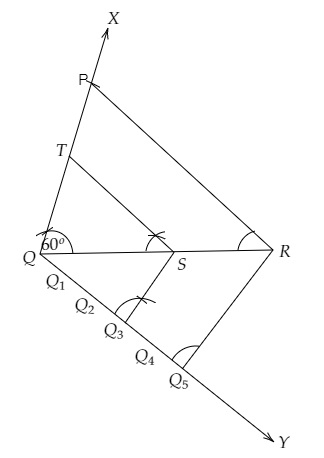# Construct a triangle $P Q R$ with side $Q R=7 \mathrm{~cm}, P Q=6 \mathrm{~cm}$ and $\angle P Q R=60^{\circ}$. Then construct another triangle whose sides are $3 / 5$ of the corresponding sides of $\triangle P Q R$.

Given:

A triangle $P Q R$ with side $Q R=7 \mathrm{~cm}, P Q=6 \mathrm{~cm}$ and $\angle P Q R=60^{\circ}$.

To do:

We have to construct a triangle $P Q R$ with side $Q R=7 \mathrm{~cm}, P Q=6 \mathrm{~cm}$ and $\angle P Q R=60^{\circ}$. Then construct another triangle whose sides are $3 / 5$ of the corresponding sides of $\triangle P Q R$.

Solution:Steps of construction:

(i) Draw a line segment $QR = 7\ cm$.

(ii) At $Q$ draw a ray $QX$ making an angle of $60^o$ and cut off $PQ = 6\ cm$. Join $PR$.

(iii) Draw a ray $QY$ making an acute angle and cut off five equal parts.

(iv) Join $Q_5R$ and through $Q_3$, draw $Q_3S$ parallel to $Q_5R$ which meets $QR$ at $S$.

(v) Through $S$, draw $ST\ \parallel\ RP$ meeting $PQ$ at $T$.

$QST$ is the required triangle.